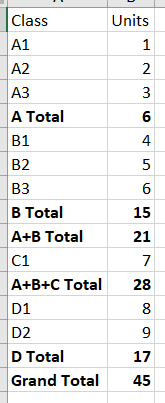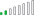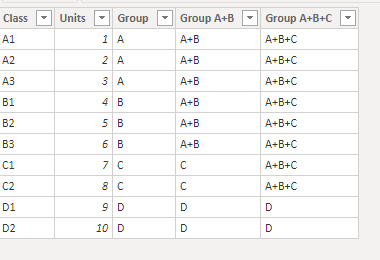cancel
Showing results for
Did you mean:Microsoft

## Multiple sub totals in a single table or matrix

Team,

Trying to learn a measure or way by which I can have a table or matrix in Power BI sub total as follows:

Right now, in a single table, I can get A, B, C, D, and grand total to show...  but not A+B or A+B+C.

Best,

mibu2 ACCEPTED SOLUTIONSMicrosoft

This could be done with calculation groups, adapting this approach (see the 2nd part) on asymmetric matrix.

Building a Matrix with Asymmetrical Columns and Rows in Power BI - Excelerator BI

Pat

Did I answer your question? Mark my post as a solution! Kudos are also appreciated!Resolver II

You need to create hierarchies by adding columns then use them in the matrix.4 REPLIES 4Resolver II

You need to create hierarchies by adding columns then use them in the matrix.Microsoft

This did the trick.  Thank you!  🙂Resolver II

You're Welcome 🙂Microsoft

This could be done with calculation groups, adapting this approach (see the 2nd part) on asymmetric matrix.

Building a Matrix with Asymmetrical Columns and Rows in Power BI - Excelerator BI

Pat

Did I answer your question? Mark my post as a solution! Kudos are also appreciated!